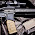## onsdag 22 november 2017

### Resetting reset handling

I will make a bold statement. You are probably doing your reset wrong in your RTL code. Yep, that's right. There are of course plenty of ways to resetting things the wrong way. Asynchronous vs synchronous is one example. Active high or active low is another.

But that's not why we're here today. Instead I will talk about a bad coding style that is so prevalent that I feel it needs some mentioning. Consider the following verilog code of a component that is most likely pretty worthless in practice, but will serve well as our example

```module rst_all
(input      clk,
input      rst,
input      valid_i,
input [3:0]      data_i,
output reg      valid_o,
output reg [3:0] data_o);

reg [2:0]  count;
reg [3:0]  data_r;

always @(posedge clk) begin
if (rst) begin
data_o  <= 4'd0;
data_r  <= 4'd0;
valid_o <= 1'b0;
count   <= 3'd0;
end else begin
if (valid_i)
count <= count + 1;
data_r  <= data_i;
data_o  <= data_r + count;
valid_o <= valid_i;
end
end
endmodule
```

All our registers are being reset, as can be seen in the following screenshot from the elaborated code in Vivado.

Now, as good RTL designers we want to minimize the load on the reset network, so we start looking for things that don't really need to be reset. Both data_o and data_r are unnecessary to reset if we only look at the data when valid_o is asserted. Let's remove them and try again

```module rst_bad
(input clk,
input     rst,
input     valid_i,
input [3:0]     data_i,
output reg    valid_o,
output reg [3:0] data_o);

reg [2:0]  count;
reg [3:0]  data_r;

always @(posedge clk) begin
if (rst) begin
//data_o  <= 4'd0;
//data_r  <= 4'd0;
valid_o <= 1'b0;
count   <= 3'd0;
end else begin
if (valid_i)
count <= count + 1;
data_r  <= data_i;
data_o  <= data_r + count;
valid_o <= valid_i;
end
end
endmodule
```

Whoa.. what just happened The reset inputs are gone, but we suddenly have two new muxes in the design and clock enable pins on the flip flops. And what's worse, we still have the same load on the reset net. How is this possible?!?! Well, the thing is that we haven't specified what to do with the data_r and data_o signals when rst is low. Therefore, according to verilog rules (the same thing applies to VHDL designs too), we must keep the old value. This is most likely not what we wanted to do. Still, I see this design pattern all the time, and no one is warning about it. What should we do then? One way is to replicate the assignments to data_r and data_o also in the reset section, but that's pretty awkward. The real solution is much simpler, but will probably cause heart attacks among conservative RTL designers.

We put the reset handling at the end of the process. Oh yes, I just did! And no one can stop me! It looks like this by the way

```module rst_good
(input clk,
input     rst,
input     valid_i,
input [3:0]     data_i,
output reg    valid_o,
output reg [3:0] data_o);

reg [2:0]  count;
reg [3:0]  data_r;

always @(posedge clk) begin
begin
if (valid_i)
count <= count + 1;
data_r  <= data_i;
data_o  <= data_r + count;
valid_o <= valid_i;

end
if (rst) begin
//data_o  <= 4'd0;
//data_r  <= 4'd0;
valid_o <= 1'b0;
count   <= 3'd0;
end
end
endmodule
```

and the generated design looks like this.

Problem solved!

A few additional notes:
1. I have put together a FuseSoC core with the source and some notes on how to simulate and build the designs mentioned here in a git repo
2. This was an example with synchronous resets, but the same thing is true for asynchronous.
3. I have no idea why Vivado chooses to instantiate the muxes however, as it could just use the CE port directly. Other tools do that. My guess is that CE maybe is always active high, and the mux serves as an inverter.
4. If you only target FPGA and the reset is just a power-on-reset, you don't need to reset at all. Just provide an init value if you must. Don't be afraid. It works, and will save some load on your reset net.
5. There are many many more things to say about resets. But we stop here for today

#### 5 kommentarer:

1.As an alternative (and my preference) you can put rst check still first but assign 4'dx to data_o and data_r. Quick check with yosys seems to give same wanted result.

1.I heard the same thing from another reader just two days ago :) Had no idea about that method before. I tried it in Vivado after that, and it's the same result there too. It's a good technique to know about as it minimizes the differences between resetting and not resetting. It could potentially be used with a `define to select this at compile-time.

It doesn't help however with the (in my experience quite common) case where you simply forget to add a signal to the reset clause that you use in the process

2.gracias por compartir la información, thanks for your information

3.what if both reset and valid_i are high at same time ?

1.The last statement will be performed, so count and valid_o will be reset :)
/Bjorn